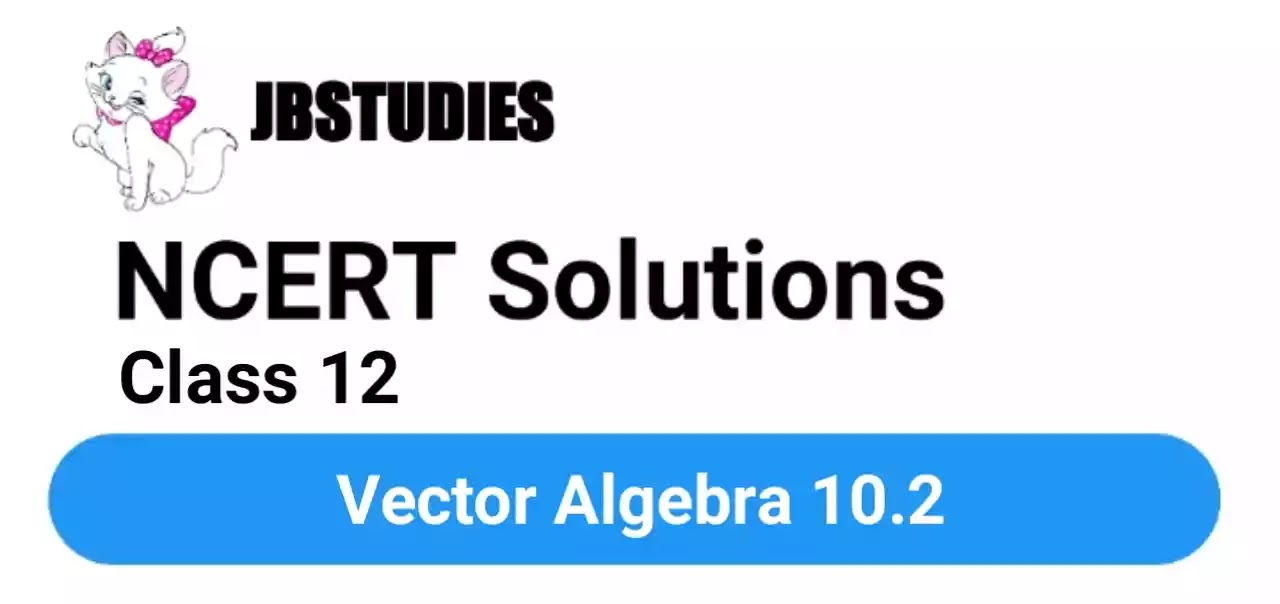# NCERT Solutions Class 12 Maths Chapter-10 (Vector Algebra)Exercise 10.2

NCERT Solutions Class 12 Maths from class 12th Students will get the answers of Chapter-10 (Vector Algebra)Exercise 10.2 This chapter will help you to learn the basics and you should expect at least one question in your exam from this chapter.
We have given the answers of all the questions of NCERT Board Mathematics Textbook in very easy language, which will be very easy for the students to understand and remember so that you can pass with good marks in your examination.### Exercise 10.2

Q1. Compute the magnitude of the following vectors:

Answer. The given vectors are: $\stackrel{\to }{a}=\stackrel{^}{i}+\stackrel{^}{j}+\stackrel{^}{k};\phantom{\rule{1em}{0ex}}\stackrel{\to }{b}=2\stackrel{^}{i}-7\stackrel{^}{j}-3\stackrel{^}{k};\phantom{\rule{1em}{0ex}}\stackrel{\to }{c}=\frac{1}{\sqrt{3}}\stackrel{^}{i}+\frac{1}{\sqrt{3}}\stackrel{^}{j}-\frac{1}{\sqrt{3}}\stackrel{^}{k}$ $\begin{array}{rl}|\stackrel{\to }{a}|& =\sqrt{\left(1{\right)}^{2}+\left(1{\right)}^{2}+\left(1{\right)}^{2}}=\sqrt{3}\\ |\stackrel{\to }{b}|& =\sqrt{\left(2{\right)}^{2}+\left(-7{\right)}^{2}+\left(-3{\right)}^{2}}\\ & =\sqrt{4+49+9}\\ & =\sqrt{62}\end{array}$

Q2. Write two different vectors having same magnitude.

Answer. $\begin{array}{}\end{array}$ Consider a=(i^2j^+3k^) and b=(2i^+j^3k^) It can be observed that |a|=12+(2)2+32=1+4+9=14 and

Q3. Write two different vectors having same direction.

Answer. $\begin{array}{}\end{array}$ Consider p=(i^+j^+k^) and q=(2i^+2j^+2k^) The direction cosines of p are given by,

Q4. Find the values of  are equal.

Answer. The two vector  will be equal if their corresponding components are equal. Hence, the required values of x and y are 2 and 3 respectively.

Q5. Find the scalar and vector components of the vector with initial point (2, 1) and terminal point( -5, 7).

Answer. The vector with the initial point p (2, 1) and terminal point Q ( 5, 7) can be given by, $\begin{array}{l}\stackrel{\to }{\mathrm{P}\mathrm{Q}}=\left(-5-2\right)\stackrel{^}{i}+\left(7-1\right)\stackrel{^}{j}\\ ⇒\stackrel{\to }{\mathrm{P}\mathrm{Q}}=-7\stackrel{^}{i}+6\stackrel{^}{j}\end{array}$ Hence, the required scalar components are —7 and 6 while the vector components are

Q6. Find the sum of the vectors

Answer. The given vector are

Q7. Find the unit vector in the direction of the vector

Q8. Find the unit vector in the direction of vector $\stackrel{\to }{PQ}$ , where p and Q are the points (1, 2, 3) and (4, S, 6), respectively.

Q10. Find a vector in the direction of vector $5\stackrel{^}{i}-\stackrel{^}{j}+2\stackrel{^}{k}$ which has magnitude 8 units.